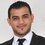# $\frac{\sin(nx)}{\sin(x)}$

\begin{align} \frac{\sin(nx)}{\sin(x)} &= \frac{e^{inx}-e^{-inx}}{e^{ix}-e^{-ix}} = \frac{e^{inx}}{e^{ix}} \cdot \frac{e^{-2inx}-1}{e^{-2ix}-1} & {\color{red} \sin(\theta) = \frac{e^{i\theta}-e^{-i\theta}}{2i} }\\ &= \frac{e^{inx}}{e^{ix}} \cdot \sum_{k=0}^{n-1} \left (e^{-2ix} \right )^k = e^{ix(n-1)} \cdot \sum_{k=0}^{n-1} e^{-2ixk}=\sum_{k=0}^{n-1} e^{ix(n-1-2k)} & {\color{red} \sum_{k=0}^{n-1} x^k = \frac{x^n - 1}{x-1}} \\ &= \underbrace{e^{ix(n-1)}+e^{ix(n-3)} +... {\color{blue} + e^{-ix(n-3)} + e^{-ix(n-1)}}}_{n \ term} \\ &= \left\{\begin{matrix} e^{ix(n-1)}+e^{ix(n-3)}+ \dots+e^{4ix}+e^{2ix}+\underbrace{e^0}_{k=\frac{n-1}{2}} + {\color{blue} e^{-2ix}+e^{-4ix} \dots + e^{-ix(n-3)} + e^{-ix(n-1)}} & n \ is \ odd\\ e^{ix(n-1)}+e^{ix(n-3)}+ \dots+e^{3ix}+e^{ix} + {\color{blue} e^{-ix}+e^{-3ix}\dots+ e^{-ix(n-3)} + e^{-ix(n-1)}} & n \ is \ even \end{matrix}\right.\\ &= \left\{\begin{matrix} 1+\sum_{k=1}^\frac{n-1}{2} {\color{red} 2} \cdot \frac{e^{2k ix}+e^{-2k ix}}{\color{red} 2} & n \ is \ odd\\ \sum_{k=1}^\frac{n}{2} {\color{red} 2} \cdot \frac{e^{(2k-1) ix}+e^{-(2k-1) ix}}{\color{red} 2} & n \ is \ even \end{matrix}\right.\\ &= \left\{\begin{matrix} 1+2 \sum_{k=1}^\frac{n-1}{2} \cos(2kx) & n \ is \ odd\\ 2 \sum_{k=1}^\frac{n}{2} \cos\left ( (2k-1)x \right ) & n \ is \ even \end{matrix}\right. \end{align}Note by Hassan Abdulla
9 months, 3 weeks ago

This discussion board is a place to discuss our Daily Challenges and the math and science related to those challenges. Explanations are more than just a solution — they should explain the steps and thinking strategies that you used to obtain the solution. Comments should further the discussion of math and science.

When posting on Brilliant:

• Use the emojis to react to an explanation, whether you're congratulating a job well done , or just really confused .
• Ask specific questions about the challenge or the steps in somebody's explanation. Well-posed questions can add a lot to the discussion, but posting "I don't understand!" doesn't help anyone.
• Try to contribute something new to the discussion, whether it is an extension, generalization or other idea related to the challenge.
• Stay on topic — we're all here to learn more about math and science, not to hear about your favorite get-rich-quick scheme or current world events.

MarkdownAppears as
*italics* or _italics_ italics
**bold** or __bold__ bold
- bulleted- list
• bulleted
• list
1. numbered2. list
1. numbered
2. list
Note: you must add a full line of space before and after lists for them to show up correctly
paragraph 1paragraph 2

paragraph 1

paragraph 2

[example link](https://brilliant.org)example link
> This is a quote
This is a quote
    # I indented these lines
# 4 spaces, and now they show
# up as a code block.

print "hello world"
# I indented these lines
# 4 spaces, and now they show
# up as a code block.

print "hello world"
MathAppears as
Remember to wrap math in $$ ... $$ or $ ... $ to ensure proper formatting.
2 \times 3 $2 \times 3$
2^{34} $2^{34}$
a_{i-1} $a_{i-1}$
\frac{2}{3} $\frac{2}{3}$
\sqrt{2} $\sqrt{2}$
\sum_{i=1}^3 $\sum_{i=1}^3$
\sin \theta $\sin \theta$
\boxed{123} $\boxed{123}$

## Comments

Sort by:

Top Newest

Relevant: Dirichlet kernel.

- 9 months, 3 weeks ago

Log in to reply

Can we generalise this to non integral values of $n$ ? What if we wanted to find $\int_{0}^{\pi}\frac{\sin\left(xy\right)}{\sin x}dx$ when $y$ varies over the reals greater than $2$ ? @Pi Han Goh @Hassan Abdulla

- 9 months, 3 weeks ago

Log in to reply

If $y$ is not an integer, then the integrand (might) diverge at $x=\pi$.

- 9 months, 3 weeks ago

Log in to reply

×

Problem Loading...

Note Loading...

Set Loading...